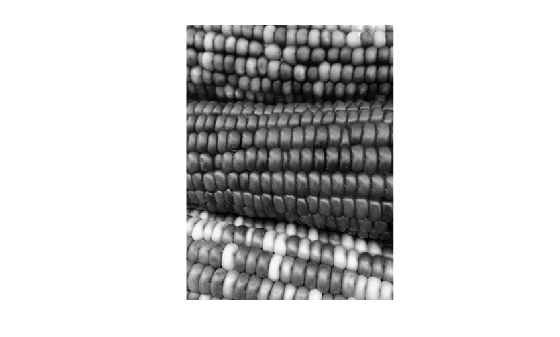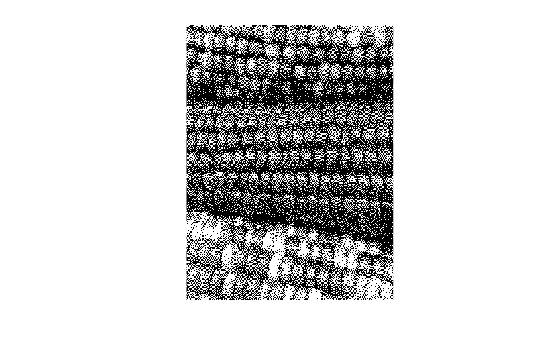dither

Convert image, increasing apparent color resolution by dithering

Description

X = dither(RGB,map) creates an indexed image approximation of the RGB image by dithering the colors in the colormap map.

X = dither(RGB,map,Qm,Qe) also specifies the number Qm of quantization bits to use along each color axis for the inverse colormap, and the number Qe of quantization bits to use for the color space error calculations.

example

BW = dither(I) converts the grayscale image I to the binary (black and white) image BW by dithering.

Examples

collapse all

Read the grayscale image from the corn.tif file into the MATLAB® workspace. The grayscale version of the image is the third image in the file.

Display the grayscale image using imshow.

imshow(corn_gray)Convert the image to binary by using the dither function.

corn_bw = dither(corn_gray);

Display the binary image. Although pixels in the binary image only have the value 0 or 1, the image appears to have shades of gray because of dithering.

imshow(corn_bw)Input Arguments

collapse all

RGB image, specified as an m-by-n-by-3 array of nonnegative numbers. If you specify an image of data type double, then values must be in the range [0, 1].

Data Types: single | double | uint8 | uint16

Input colormap, specified as a c-by-3 matrix with values in the range [0, 1]. Each row of map is a three-element RGB triplet that specifies the red, green, and blue components of a single color of the colormap. The colormap has a maximum of 65,536 colors.

Data Types: double

Quantization bits along each color axis for the inverse colormap, specified as a positive integer. If Qe is less than Qm, then dithering cannot be performed and the dither function returns an undithered indexed image in X.

Data Types: double

Quantization bits for the color space error calculations, specified as a positive integer. If Qe is less than Qm, then dithering cannot be performed and the dither function returns an undithered indexed image in X.

Data Types: double

Grayscale image, specified as an m-by-n matrix of nonnegative numbers. If you specify an image of data type double, then values must be in the range [0, 1].

Data Types: single | double | int16 | uint8 | uint16

Output Arguments

collapse all

Indexed image, returned as an m-by-n matrix of nonnegative integers. If the length of map is less than or equal to 256, then the output image is of class uint8. Otherwise, the output image is of class uint16. The value 0 in the output array X corresponds to the first color in the colormap.

Note

The values in image X are indexes into the colormap map and should not be used in mathematical processing, such as filtering operations.

Data Types: uint8 | uint16

Binary image, returned as an m-by-n logical matrix.

Data Types: logical

Algorithms

dither increases the apparent color resolution of an image by applying Floyd-Steinberg's error diffusion dither algorithm .

 Floyd, R. W., and L. Steinberg, "An Adaptive Algorithm for Spatial Gray Scale," International Symposium Digest of Technical Papers, Society for Information Displays, 1975, p. 36.

 Lim, Jae S., Two-Dimensional Signal and Image Processing, Englewood Cliffs, NJ, Prentice Hall, 1990, pp. 469–476.